## General Question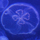# Calculus homework help!!!

Asked by luv2mahsky (5) February 17th, 2008

A cigarette contains about 0.4 mg of nicotine. the half-life of nicotine is about 2 hours.

A. What is the hourly rate of decay of nicotine?

B. what is the amount of nicotine in the body 24 hours after a cigarette was smoked ( assuming only one was smoked in that time interval)

C. How long does it take for the level of nicotine ina smoker’s body to be ruduced to .08 mg?

D. How long does it take for the level of nicotine in the body to be reduced by 20%?

Observing members: 0Composing members: 0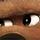Hi Meredith. Pay attention in class from now on, thanks :P

PS I’m coming up to the room in like… an hour.

chad (694)“Great Answer” (0) Flag as…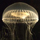hi luv2mahsky,

This looks like a deriviative problem to me. I would find dm/dt first where m is mass of nicotine with respect to time (t)

Knowing that should answer b and c and a pathway to the rest of the problem. a good place for some info is mathforum.com

Hope it helps

artur (21)“Great Answer” (1) Flag as…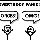If the half life is 2 hours, that means that in two hours you will have half of what you originally started with. You start with assuming a exponential decay relationship, i.e. Mass = Mass_original * e^(rt), where r is the rate constant. Because you know that when t = 2 the Mass left will be half of the original mass, regardless of how much you started with, (Mass_original / 2) = Mass_original * e^(2r). Solving the equation for r (see how Mass_original drops out?), r = (ln2)/2. You then have the exponential rate equation, m = m_o*e^(rt). This can also be expressed in the form m = m_0 * a^t, where a = e^r.

As for your four questions, once you have the equation they can be solved as follows.
1. plug in t = 1 and solve for m/m_o
2. plug in .4 for m_o and 24 for t (assuming instantaneous cigarette smoking at t = 0), solve for m.
3. plug in .4 for m_o and .08 for m, solve for t
4. solve general equation for m/m_o, plug in .2 for m/m_o, solve for t.

Hope this helps in time!

jdvanderk (155)“Great Answer” (1) Flag as…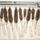This seems a little like cheating to me. Am I off base here? Maybe it is because I didn’t have daily access to the internet when I took calculus.

wabarr (458)“Great Answer” (0) Flag as…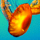This is exponential decay. Look it up.

No more help – that’s cheating!

Kurtosis (95)“Great Answer” (0) Flag as…Response moderated (Personal Attack)

or